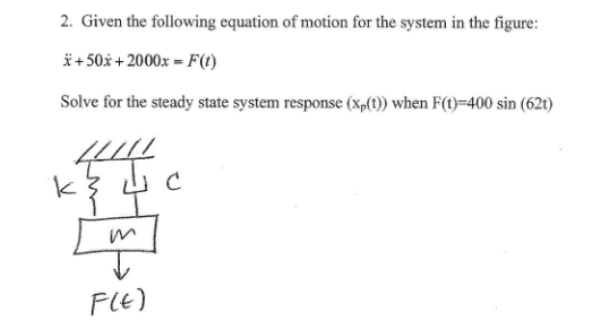Given the following equation of motion for the system in the figure: xdoubdot + 50 xdot + 2000x = F(t) , solve for the steady state system response (Xp(t)) when F(t)=400 sin(62t).Given the following equation of motion for the system in the figure: xdoubdot + 50 xdot + 2000x = F(t) , solve for the steady state system response (Xp(t)) when F(t)=400 sin(62t).

Vibrations Page 1 vibrations vibrations vibrations vibrations vibrations vibrations vibrations Vibrations Page 2